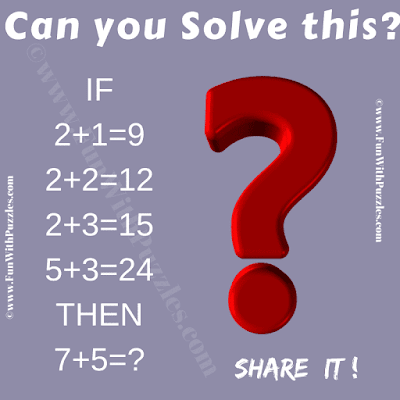It is a very interesting crack the code for high school students. Solving this puzzle will help students to test their logical and mathematical skills. In this code-cracking puzzle, you are shown some logical number equations. These equations follow some logical or mathematical code. Your challenge is to crack the code and find the value of the missing number which will replace the question mark in the last equation. Can you crack the code?Can you Crack the Code?

The answer to this "Crack the Code Puzzle for Students", can be viewed by clicking on the answer button.